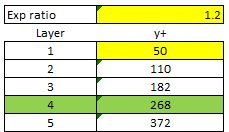# Mesh Independence Study - Layering disappears across study meshes

#41

@DaleKramer

I hope you get the results that you want. But bear in mind that there is another side of CFD - discretization schemes and linear solver settings. If you still run into problems, share your project here so I can have a look.

#42

Look out, I plan to take you up on that offer at some pointDale

#43

Before you leave, I am a little unsure of what you mean here. Are you talking the plane surface refinement levels ‘normal’ to flow dimension or ‘along’ the flow dimension?

Dale

#44

@DaleKramer

Sorry my bad. In the refinement settings, you can define refinement levels on the fuselage, the wings, and landing gears, etc. You can also define refinement levels on a box that covers the plane, so that the cells within the box will have at least that refinement level. For example, with a background mesh of 0.5m generated from blockMesh, you can define level 8 refinement on the plane, and level 6 refinement on a box that covers the plane. Now, if you define a level 9 refinement on the box, then the entire plane will also have level 9 refinement, finer than what you need creating a lot of cells.

When doing mesh independency study, you can increase the level of refinement of that box to 7, but you don’t need to increase the level on the plane. If there are areas on the plane that are apparently not described by the surface mesh properly, then you refine it.

#45

Ok, so would you consider these ‘space’ refinement levels sufficient for the 15 layer boundary?

#46

Yes, I think the space refinement is enough. However, you want to increase a parameter called ‘nCellsBetweenLevels’ to 4 or 5 so that the transition between levels is smoother. At the moment it seems to be 2 or 3. Also, you don’t want 15 layers in this case, because:

1. using an averaged y+ = 50 first layer, with a growth rate of 1.2, 4 of these layers will yield an overal y+ = 50+50 * 1.2+50 * 1.2 * 1.2+50 * 1.2 * 1.2 * 1.2 = 268.4. A turbulent boundary layer ranges from y+ = 100 to 300. I think 4~5 layers is sufficient.
2. even with 15 layers, the transition between the final layer and the adjacent cell is still abrupt – very large volume size change between the two. You want to avoid such behaviour.

#47

Sorry, I used that previous image only to see what kind of space refinement levels were required with respect to the final BL layer. That is not how I have layered my yPlus 30-100 mesh. The 30-100 mesh only has 3 layers like this:

Is this better?

Dale

#48

@DaleKramer

That looks much better.

#49I think I’m off to the races now …

#50

Hi Dylan, @dylan

Could you explain how your formula, that calculates a yPlus value for the 4th boundary layer, was derived and what purpose does it serve?

Is it somehow a guide for how many layers to choose based on a particular 1st layer y+ value and layer expansion ratio?

If that is the case, is this layering setup somehow a most optimized setup for a desired 1st layer y+ and layer expansion ratio?

Your formula seems to determine the yPlus value of each layer ( YP(x) ), and that value is based on the 1st layer yPlus value ( YP(1) ) and an exponential progression of the expansion ratio (ER). The formula can be summarized as:

YP(x) = YP(x-1) + YP(1)*ER^YP(x)

(derived from your equation for the 4th layer y+ = 50+50 * 1.2+50 * 1.2 * 1.2+50 * 1.2 * 1.2 * 1.2 = 268.4)

With 1.2 ER and a 1st layer y+ of 50, here is the progression of your formula:I thought a desired yPlus was used in the online yPlus calculator in order to determine ONLY the 1st layer thickness.

I can not find any information of yPlus values being assigned to any layer but the first Layer.

When I view my yPlus values of a converged simulation, they are only on the geometry surface, the 2nd and further away layers are mapped at yPlus=0, like this 6 layer BL:

I am confusedThanks,
Dale